# 5. -18.33 points Consider the following matrix, A. For what value of k will Al-20? 6....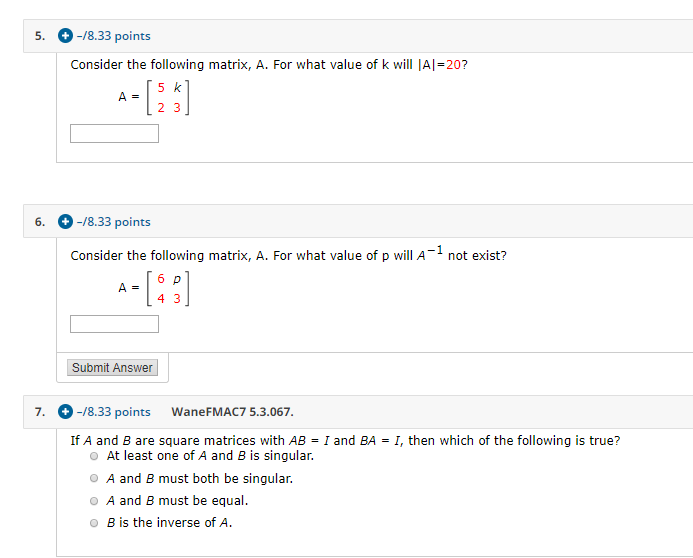5. -18.33 points Consider the following matrix, A. For what value of k will Al-20? 6. -18.33 points Consider the following matrix, A. For what value of p will A-1 not exist? Submit Answer 7. -18.33 points WaneFMAC7 5.3.067. If A and B are square matrices with AB = I and BA = I, then which of the following is true? At least one of A and B is singular. O A and B must both be singular. O A and B must be equal. OB is the inverse of A.

(5)

Determinant of Matrix A = (5 X 3) - (2 X k) = 15 - 2k

Given:

Determinant of Matrix A = 20

So,

15 - 2k = 20

So,

2k = 15 - 20 = - 5

So,

k = - 2.5

So,

- 2.5

(b)

A-1 will not exist if determinant of A = 0

Determinant of matrix A = (6 X 3) - (4 X p)

= 18 - 4p

Thus,

A-1 will not exist if 18 - 4p = 0

i.e.,

p = 18/4 = 4.5

So,

4.5

(c)

Correct option:

B is the inverse of A

#### Earn Coin

Coins can be redeemed for fabulous gifts.

Similar Homework Help Questions
• ### 15, a (2 points) Consider the matrix, A = For what value offwill A1 i.e. Inverse...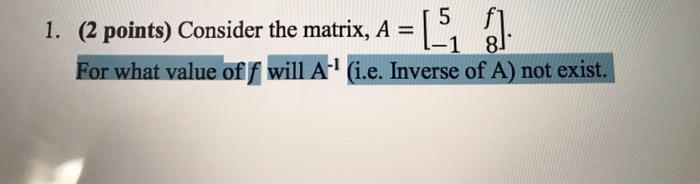15, a (2 points) Consider the matrix, A = For what value offwill A1 i.e. Inverse of A) not exist. I. 1 8

• ### Problem 4 (12 points, 2 for each part) In each of the following parts, as- sume...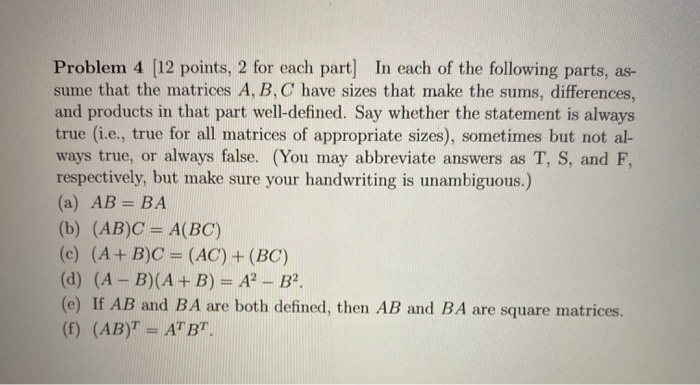Problem 4 (12 points, 2 for each part) In each of the following parts, as- sume that the matrices A, B, C have sizes that make the sums, differences, and products in that part well-defined. Say whether the statement is always true (i.e., true for all matrices of appropriate sizes), sometimes but not al- ways true, or always false. (You may abbreviate answers as T, S, and F, respectively, but make sure your handwriting is unambiguous.) (a) AB = BA...

• ### L. Answer True or False. Justify your answer (a) Every linear system consisting of 2 equations...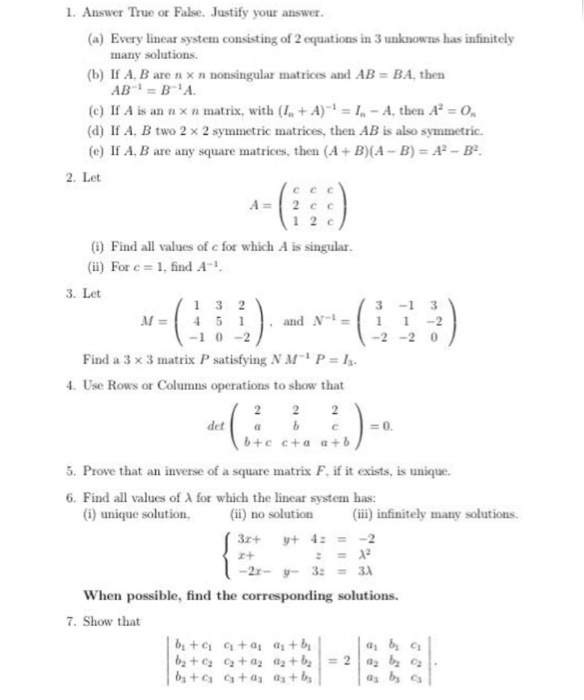L. Answer True or False. Justify your answer (a) Every linear system consisting of 2 equations in 3 unknowns has infinitely many solutions (b) If A. B are n × n nonsingular matrices and AB BA, then (e) If A is an n x n matrix, with ( +A) I-A, then A O (d) If A, B two 2 x 2 symmetric matrices, then AB is also symmetric. (e) If A. B are any square matrices, then (A+ B)(A-B)-A2-B2 2....

• ### (10 points)The trace of a square nxn matrix is A, denoted tr(A), is the sum of...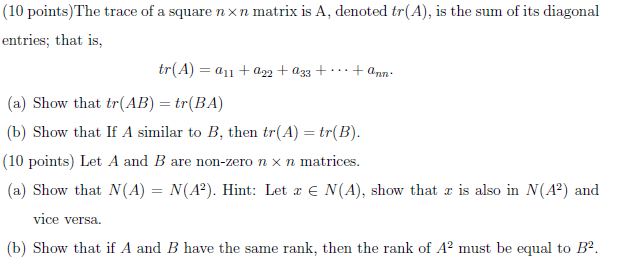(10 points)The trace of a square nxn matrix is A, denoted tr(A), is the sum of its diagonal entries; that is, tr(A) = a11+2)2 +433 +: ... + ann (a) Show that tr(AB) = tr(BA) (b) Show that If A similar to B, then tr(A) = tr(B). (10 points) Let A and B are non-zero n x n matrices. (a) Show that N(A) = N(A2). Hint: Let 2 EN(A), show that is also in N(A2) and vice versa. (b) Show...

• ### 1 -1 -b 1 The inverse of matrix A is (see explanation in Sec. 5.6) and...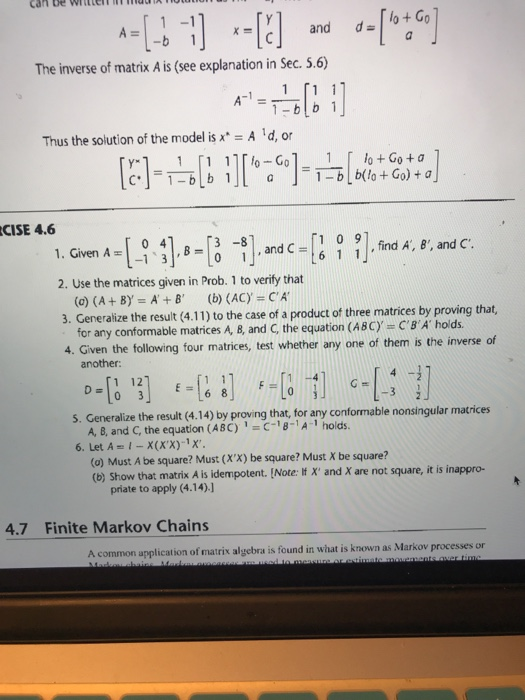1 -1 -b 1 The inverse of matrix A is (see explanation in Sec. 5.6) and d lo+Go A-1 1 1-blb 1 Thus the solution of the model isx A d, or CISE 4.6 1.Given A-B1--B -t].and c-l 1 0 9 ].find A, e-arnd C -1 3 , find A, 8', and C 2. Use the matrices given in Prob. 1 to verify that 3. Generalize the result (4.11) to the case of a product of three matrices by proving...

• ### QUESTION 3 It is possible for two functions to have a same Laplace transform True False...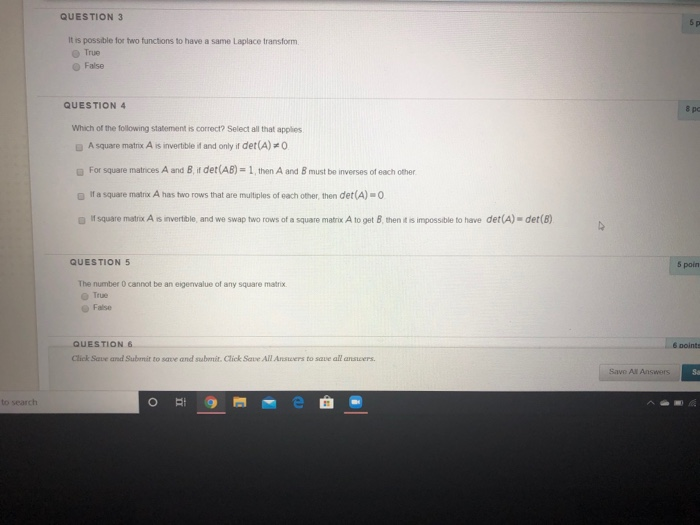QUESTION 3 It is possible for two functions to have a same Laplace transform True False QUESTION 4 Which of the following statement is correct? Select all that applies A square matrix A is invertible it and only if det(A)=0 For square matrices A and B ir det(AB) = 1 then A and B must be inverses of each other. If a square matrix A has two rows that are multiples of each other, then det(A) - 0 If square...

• ### 5. Let B be the following matrix in reduced row-echelon form: 1 B= 1 -1 0-1...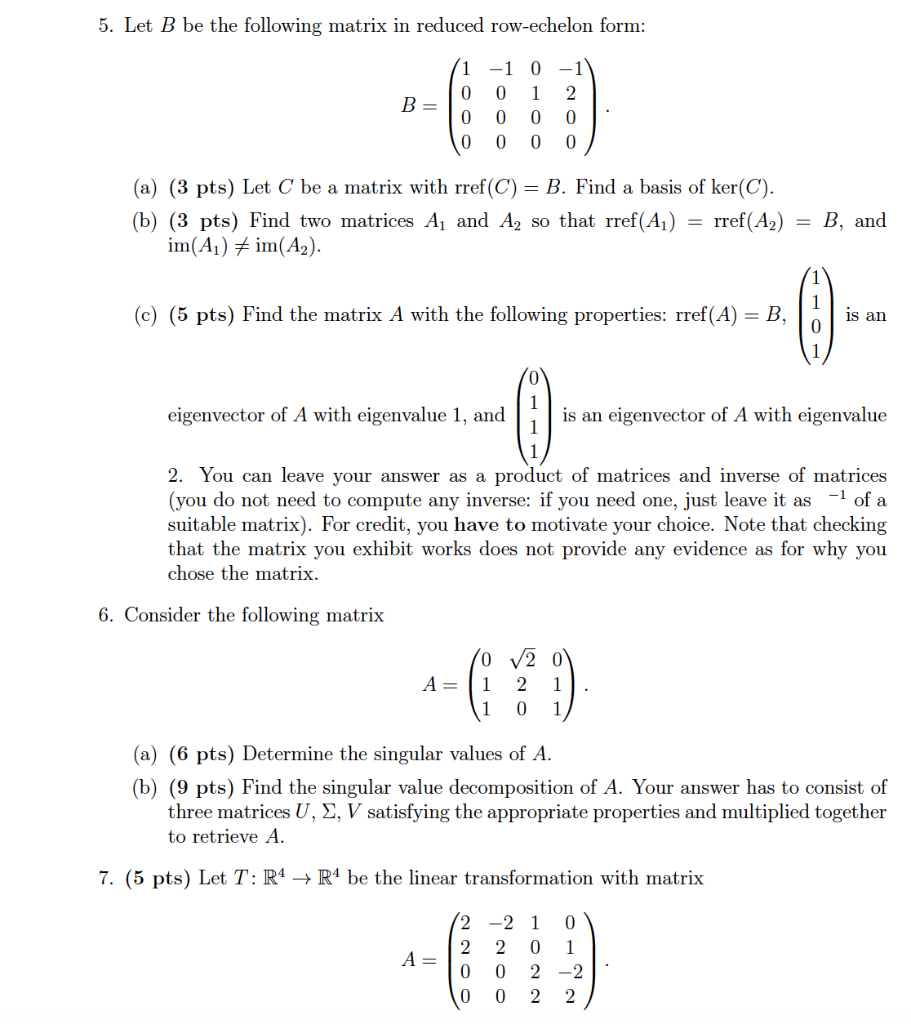5. Let B be the following matrix in reduced row-echelon form: 1 B= 1 -1 0-1 0 0 2 0 0 0 0 0 0 0 0 (a) (3 pts) Let C be a matrix with rref(C) = B. Find a basis of ker(C). (b) (3 pts) Find two matrices A1 and A2 so that rref(A1) = rref(A2) im(A) # im(A2). B, and 1 (c) (5 pts) Find the matrix A with the following properties: rref(A) = B, is an...

• ### IT a) If one row in an echelon form for an augmented matrix is [o 0 5 o 0 b) A vector bis a linear combination of the columns of a matrix A if and only if the c) The solution set of Ai-b is the set o...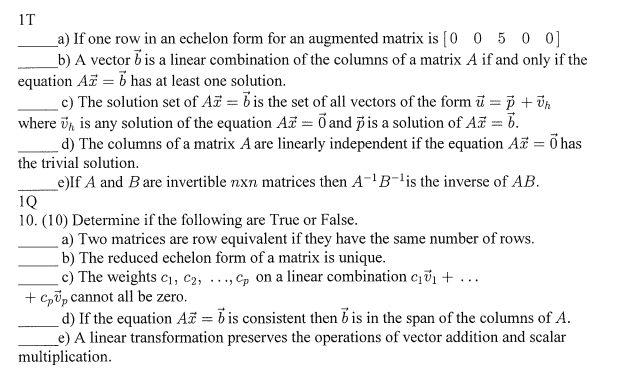IT a) If one row in an echelon form for an augmented matrix is [o 0 5 o 0 b) A vector bis a linear combination of the columns of a matrix A if and only if the c) The solution set of Ai-b is the set of all vectors of the formu +vh d) The columns of a matrix A are linearly independent if the equation A 0has If A and Bare invertible nxn matrices then A- B-'is the...

• ### That AB- 100 1 2 20 2 2 0 and trices shown to the right e A be the oorresponding n ×n matrix and ...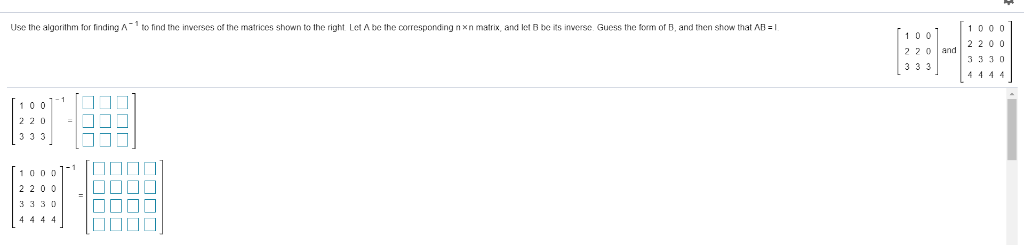that AB- 100 1 2 20 2 2 0 and trices shown to the right e A be the oorresponding n ×n matrix and let B be i s in ers e Guess the to m or B, and then st Use the algorithm for finding A to find the invers s of the 3 330 3 330 4444] 11 1[10 If A is the corresponding n× n matrix and B is its inverse, which of the following is B?...

• ### Question 1 of 8 1.0 Points 11  [o 1 1] , B= 0 1 2...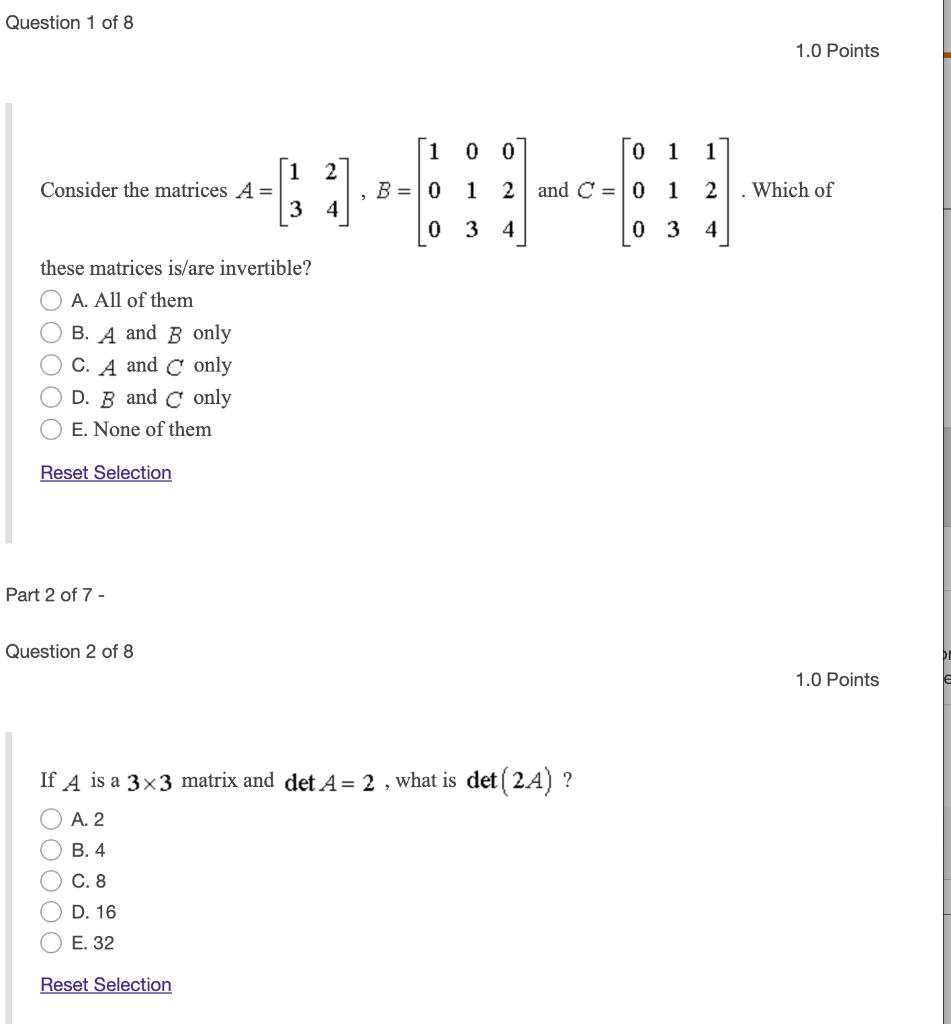Question 1 of 8 1.0 Points 11  [o 1 1] , B= 0 1 2 and C = 0 1 2. Which of 10 3 4 10 3 4] Consider the matrices A= 3 4 these matrices is/are invertible? O A. All of them O B. A and B only O C. A and C only OD. B and C only O E. None of them Reset Selection Part 2 of 7 - Question 2 of 8 1.0 Points...